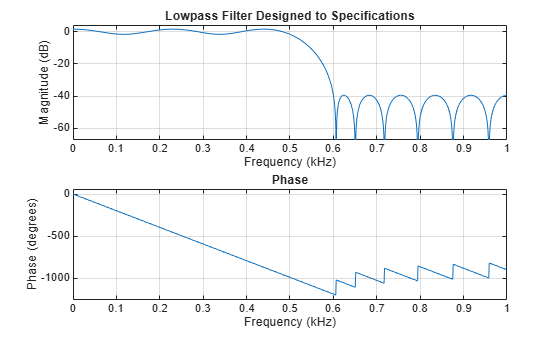# firpmord

Parks-McClellan optimal FIR filter order estimation

## Syntax

``[n,fo,ao,w] = firpmord(f,a,dev)``
``[___] = firpmord(___,fs)``
``c = firpmord(___,'cell')``

## Description

````[n,fo,ao,w] = firpmord(f,a,dev)` returns the approximate order `n`, normalized frequency band edges `fo`, frequency band amplitudes `ao`, and weights `w` that meet input specifications `f`, `a`, and `dev`.```

example

````[___] = firpmord(___,fs)` specifies a sampling frequency `fs`. `fs` defaults to 2 Hz, implying a Nyquist frequency of 1 Hz. You can specify band edges scaled to a particular application's sample rate. You can use this with any of the previous input syntaxes.```

example

````c = firpmord(___,'cell')` returns a cell array `c` whose elements are the parameters to `firpm`.```

## Examples

collapse all

Design a minimum-order lowpass filter with a 500 Hz passband cutoff frequency and 600 Hz stopband cutoff frequency. Specify a sampling frequency of 2000 Hz. Require at least 40 dB of attenuation in the stopband and less than 3 dB of ripple in the passband.

```rp = 3; % Passband ripple in dB rs = 40; % Stopband ripple in dB fs = 2000; % Sampling frequency f = [500 600]; % Cutoff frequencies a = [1 0]; % Desired amplitudes```

Convert the deviations to linear units. Design the filter and visualize its magnitude and phase responses.

```dev = [(10^(rp/20)-1)/(10^(rp/20)+1) 10^(-rs/20)]; [n,fo,ao,w] = firpmord(f,a,dev,fs); b = firpm(n,fo,ao,w); freqz(b,1,1024,fs) title('Lowpass Filter Designed to Specifications')```The filter falls slightly short of meeting the stopband attenuation and passband ripple specifications. Using `n+1` instead of `n` in the call to `firpm` achieves the desired amplitude characteristics.

Design a lowpass filter with a 1500 Hz passband cutoff frequency and 2000 Hz stopband cutoff frequency. Specify a sampling frequency of 8000 Hz. Require a maximum stopband amplitude of 0.1 and a maximum passband error (ripple) of 0.01.

```[n,fo,ao,w] = firpmord([1500 2000],[1 0],[0.01 0.1],8000); b = firpm(n,fo,ao,w);```

Obtain an equivalent result by having `firpmord` generate a cell array. Visualize the frequency response of the filter.

```c = firpmord([1500 2000],[1 0],[0.01 0.1],8000,'cell'); B = firpm(c{:}); freqz(B,1,1024,8000)```## Input Arguments

collapse all

Frequency band edges, specified as a real-valued vector. The argument must be in the range [0, Fs/2], where Fs is the Nyquist frequency. The number of elements in the vector is always a multiple of 2. The frequencies must be in increasing order.

Desired amplitudes at the points contained in `f`, specified as a vector. `f` and `a` must satisfy the condition length(f) = 2length(a)–2. The desired function is piecewise constant.

Maximum allowable deviation, specified as a vector. `dev` has the same size as `a`. It specifies the maximum allowable deviation or ripples between the frequency response and the desired amplitude of the output filter for each band.

Sample rate, specified as a real scalar.

## Output Arguments

collapse all

Filter order, returned as a positive integer.

Normalized frequency points, specified as a real-valued vector. The argument must be in the range [0, 1] , where 1 corresponds to the Nyquist frequency. The number of elements in the vector is always a multiple of 2. The frequencies must be in increasing order.

Amplitude response, returned as a real-valued vector.

Weights used to adjust the fit in each frequency band, specified as a real-valued vector. The length of `w` is half the length of `f` and `a`, so there is exactly one weight per band.

FIR filter parameters, returned as a cell array.

## Algorithms

`firpmord` uses the algorithm suggested in . This function produces inaccurate results for band edges close to either 0 or the Nyquist frequency, `fs/2`.

Note

In some cases, `firpmord` underestimates or overestimates the order `n`. If the filter does not meet the specifications, try a higher order such as `n+1` or `n+2`.

 Rabiner, Lawrence R., and Otto Herrmann. “The Predictability of Certain Optimum Finite-Impulse-Response Digital Filters.” IEEE® Transactions on Circuit Theory. Vol.  20, Number 4, 1973, pp. 401–408.

 Rabiner, Lawrence R., and Bernard Gold. Theory and Application of Digital Signal Processing. Englewood Cliffs, NJ: Prentice-Hall, 1975, pp. 156–157.